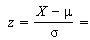# Standardized Values: Example

Hypothesis Testing > Standardized Values

Standardized values (also called standard scores or normal deviates) are the same thing as z-scores. A standardized value is what you get when you take a data point and scale it by population data. It tells us how far from the mean we are in terms of standard deviations.

How to Calculate a z-score.

Need help with a specific question? Check out our tutoring page!

## The Standardized Values Formula

If you’re asked to find standardized values, use this formula to make your calculations:You calculate a standardized value (a z-score), using the above formula. The symbols are:

These values are always given in the question.

Example question: A hot dog stand has mean daily sales of \$420 with a standard deviation of \$50. The income has a normal distribution. What is the standardized value for daily sales of \$520?

Step 1: Identify the observation (X), the mean (μ) and the standard deviation (σ) in the question.

• X = 520
• μ = 420
• σ = 50

Step 2: Plug the values from Step 1 into the formula:
Standardized value = X – μ / σ = 520 – 420 / 50.

Step 3: Use a calculator and solve:
520 – 420 / 50 = 100/50 = 2.
The standardized value is 2.

That’s it!

Tip: The question states the average (another word for the mean) and the standard deviation. But it doesn’t say “X equals”! It’s up to you to figure out the value you are finding the standardized value for. If the concept of X confused you, often it’s just as simple as using the third value given in the question.

## Example 2

Suppose your population data was 3, 3, 4, 4, and 6. The mean is 4 and the standard deviation approximately 1.2. Use the formula to standardize the data point 6:

• Subtract the mean (6 – 4 = 2),
• Divide by the standard deviation. Your standardized value (z-score) will be:
2 / 1.2 = 1.7.

The data point with value 4 has a standardized value of 4 – 4/1.2 = 0/4 = zero.

## Usefulness of Standardized Values

Standardized values are useful for tracking data that is otherwise incomparable because of different metrics or circumstances.

For instance, suppose you went to college in New York and your best friend went to college in Georgia. You might get a grade of 87 in a test with a mean of 77 and a standard deviation of 5, and the same day your friend might get a grade of 612 (mean 600, standard deviation 100). Although the two grades (87 and 612) can’t be compared directly, the standardized values will allow you to immediately see who is doing better compared with the rest of the class.

(612 – 600) / 100 = 0.12, so your friend’s z-score or standardized grade is 0.12. (87 – 77) / 5 = 2, your standardized grade. With standardized data you have grounds to boast to your friend that you are doing much better than he or she is in the class.

## Properties of Standardized Values

The mean of standardized values will always be zero, and the standard deviation will always be one. The graph of standardized values will have exactly the same shape as the graph of raw data, but it may be a different size and have different coordinates.

Check out my YouTube channel for hundreds of AP statistics and elementary statistics videos!

## References

Spiegel, Murray R.; Stephens, Larry J (2008), Schaum’s Outlines Statistics (Fourth ed.), McGraw Hill

CITE THIS AS:
Stephanie Glen. "Standardized Values: Example" From StatisticsHowTo.com: Elementary Statistics for the rest of us! https://www.statisticshowto.com/standardized-values-examples/